# 定义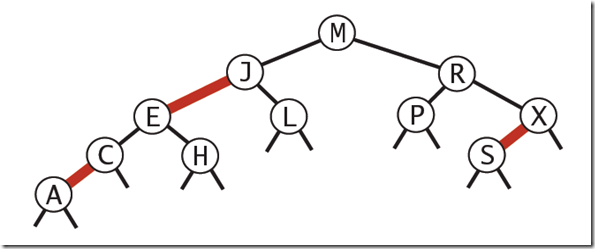• 红色节点向左倾斜
• 一个节点不可能有两个红色链接
• 整个书完全黑色平衡，即从根节点到所以叶子结点的路径上，黑色链接的个数都相同。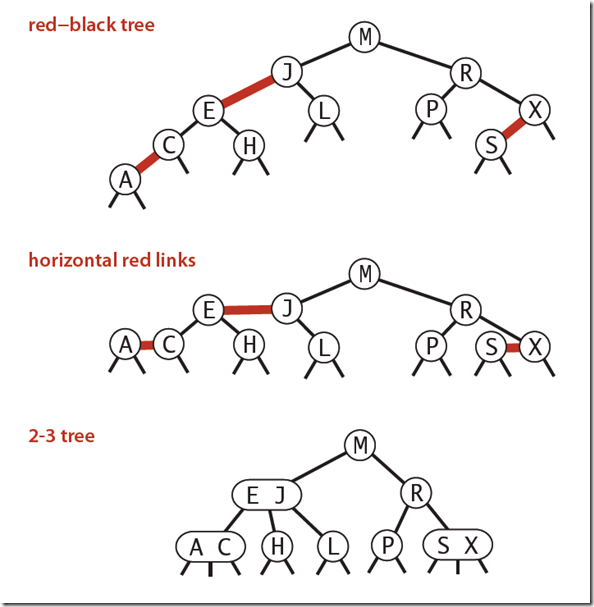# 表示

``````private const bool RED = true;
private const bool BLACK = false;

private Node root;

class Node
{
public Node Left { get; set; }
public Node Right { get; set; }
public TKey Key { get; set; }
public TValue Value { get; set; }
public int Number { get; set; }
public bool Color { get; set; }

public Node(TKey key, TValue value,int number, bool color)
{
this.Key = key;
this.Value = value;
this.Number = number;
this.Color = color;
}
}

private bool IsRed(Node node)
{
if (node == null) return false;
return node.Color == RED;
}``````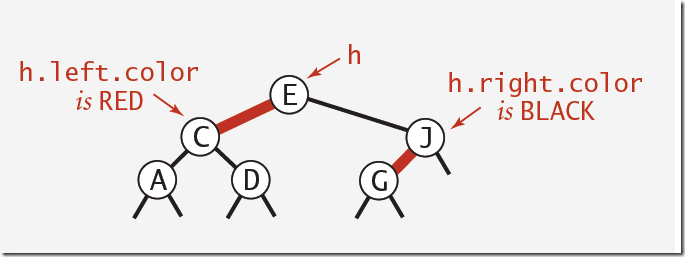# 实现

## 查找

``````//查找获取指定的值
public override TValue Get(TKey key)
{
return GetValue(root, key);
}

private TValue GetValue(Node node, TKey key)
{
if (node == null) return default(TValue);
int cmp = key.CompareTo(node.Key);
if (cmp == 0) return node.Value;
else if (cmp > 0) return GetValue(node.Right, key);
else return GetValue(node.Left, key);
}``````

## 平衡化

### 旋转

``````//左旋转
private Node RotateLeft(Node h)
{
Node x = h.Right;
//将x的左节点复制给h右节点
h.Right = x.Left;
//将h复制给x右节点
x.Left = h;
x.Color = h.Color;
h.Color = RED;
return x;
}``````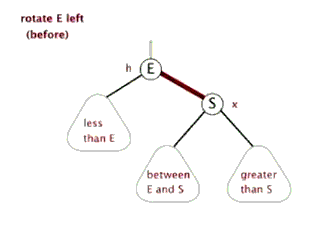``````//右旋转
private Node RotateRight(Node h)
{
Node x = h.Left;
h.Left = x.Right;
x.Right = h;

x.Color = h.Color;
h.Color = RED;
return x;
}``````Case 1 往一个2-node节点底部插入新的节点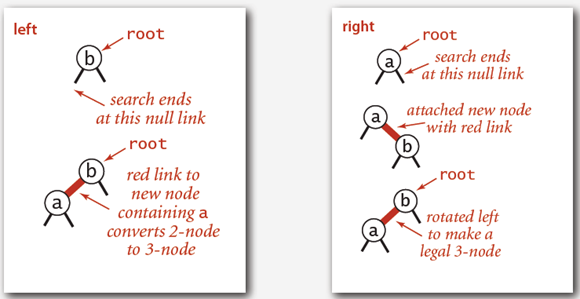• 标准的二叉查找树遍历即可。新插入的节点标记为红色
• 如果新插入的节点在父节点的右子节点，则需要进行左旋操作

Case 2往一个3-node节点底部插入新的节点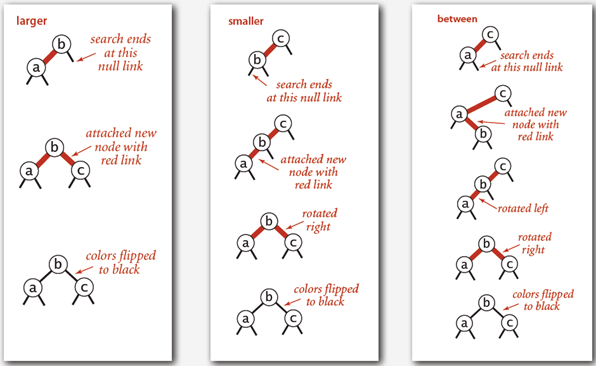• 如果带插入的节点比现有的两个节点都大，这种情况最简单。我们只需要将新插入的节点连接到右边子树上即可，然后将中间的元素提升至根节点。这样根节点的左右子树都是红色的节点了，我们只需要调研FlipColor方法即可。其他情况经过反转操作后都会和这一样。
• 如果插入的节点比最小的元素要小，那么将新节点添加到最左侧，这样就有两个连接红色的节点了，这是对中间节点进行右旋操作，使中间结点成为根节点。这是就转换到了第一种情况，这时候只需要再进行一次FlipColor操作即可。
• 如果插入的节点的值位于两个节点之间，那么将新节点插入到左侧节点的右子节点。因为该节点的右子节点是红色的，所以需要进行左旋操作。操作完之后就变成第二种情况了，再进行一次右旋，然后再调用FlipColor操作即可完成平衡操作。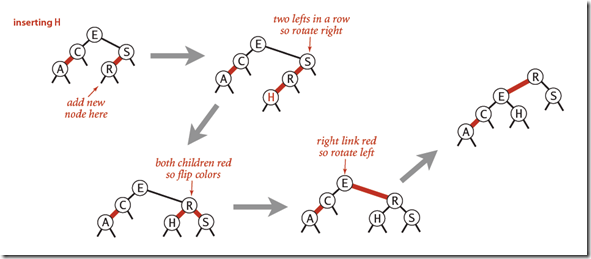1. 执行标准的二叉查找树插入操作，新插入的节点元素用红色标识。
2. 如果需要对4-node节点进行旋转操作
3. 如果需要，调用FlipColor方法将红色节点提升
4. 如果需要，左旋操作使红色节点左倾。
5. 在有些情况下，需要递归调用Case1 Case2，来进行递归操作。如下：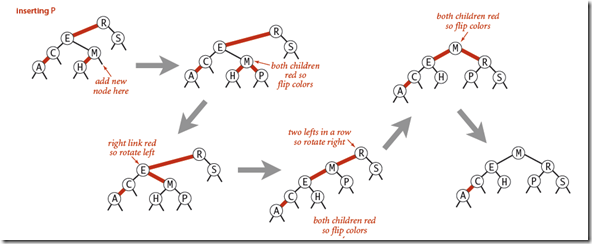## 代码实现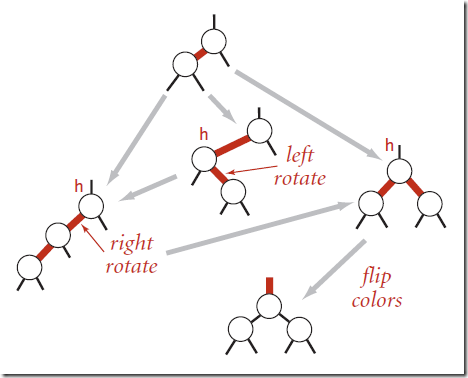• 如果节点的右子节点为红色，且左子节点位黑色，则进行左旋操作
• 如果节点的左子节点为红色，并且左子节点的左子节点也为红色，则进行右旋操作
• 如果节点的左右子节点均为红色，则执行FlipColor操作，提升中间结点。

``````public override void Put(TKey key, TValue value)
{
root = Put(root, key, value);
root.Color = BLACK;
}

private Node Put(Node h, TKey key, TValue value)
{
if (h == null) return new Node(key, value, 1, RED);
int cmp = key.CompareTo(h.Key);
if (cmp < 0) h.Left = Put(h.Left, key, value);
else if (cmp > 0) h.Right = Put(h.Right, key, value);
else h.Value = value;

//平衡化操作
if (IsRed(h.Right) && !IsRed(h.Left)) h = RotateLeft(h);
if (IsRed(h.Right) && IsRed(h.Left.Left)) h = RotateRight(h);
if (IsRed(h.Left) && IsRed(h.Right)) h = FlipColor(h);

h.Number = Size(h.Left) + Size(h.Right) + 1;
return h;
}

private int Size(Node node)
{
if (node == null) return 0;
return node.Number;
}``````

## 分析

• 以升序插入构建红黑树：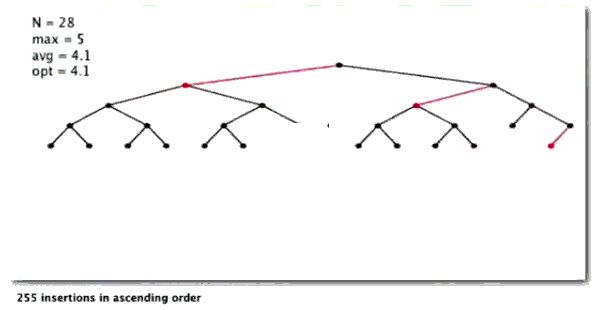• 以降序插入构建红黑树：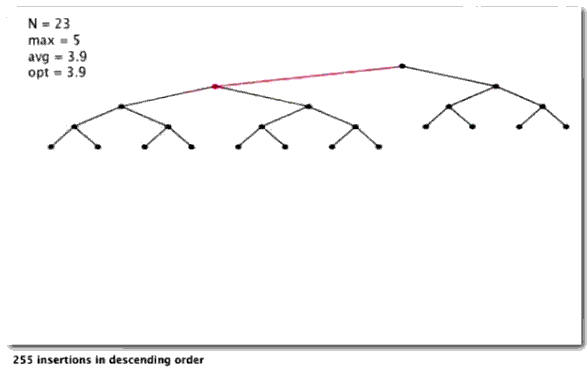• 随机插入构建红黑树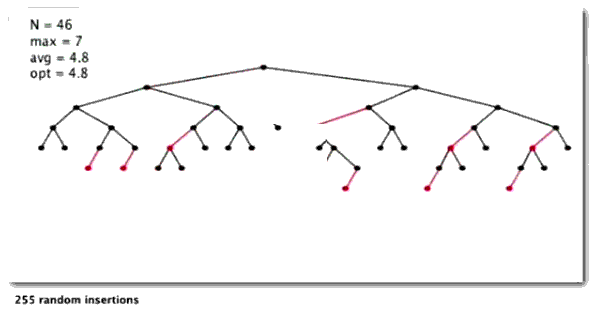## 1. 在最坏的情况下，红黑树的高度不超过2lgN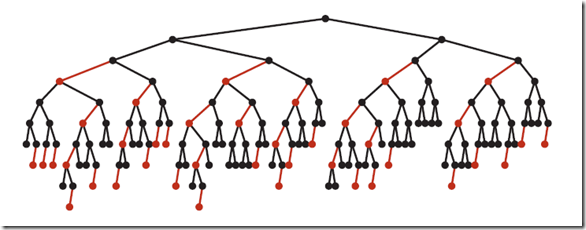## 2. 红黑树的平均高度大约为lgN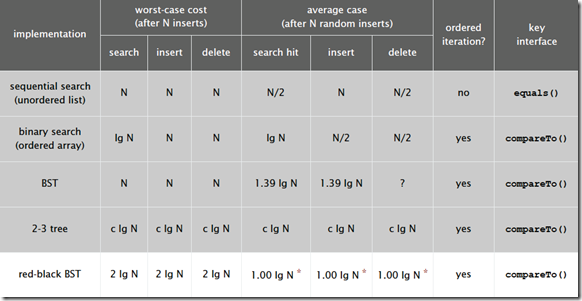# 应用

• Java中的java.util.TreeMap,java.util.TreeSet
• C++ STL中的：map,multimap,multiset
• .NET中的：SortedDictionary,SortedSet

``````public void Add(T item)
{
if (this.root == null)
{
this.root = new Node<T>(item, false);
this.count = 1;
}
else
{
Node<T> root = this.root;
Node<T> node = null;
Node<T> grandParent = null;
Node<T> greatGrandParent = null;
int num = 0;
while (root != null)
{
num = this.comparer.Compare(item, root.Item);
if (num == 0)
{
this.root.IsRed = false;
}
if (TreeSet<T>.Is4Node(root))
{
TreeSet<T>.Split4Node(root);
if (TreeSet<T>.IsRed(node))
{
this.InsertionBalance(root, ref node, grandParent, greatGrandParent);
}
}
greatGrandParent = grandParent;
grandParent = node;
node = root;
root = (num < 0) ? root.Left : root.Right;
}
Node<T> current = new Node<T>(item);
if (num > 0)
{
node.Right = current;
}
else
{
node.Left = current;
}
if (node.IsRed)
{
this.InsertionBalance(current, ref node, grandParent, greatGrandParent);
}
this.root.IsRed = false;
this.count++;
this.version++;
}
}``````

# 总结

Do you have any suggestions for improvement?